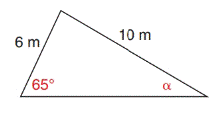Chapter 11.4, Problem 18E### Elementary Geometry for College St...

6th Edition
Daniel C. Alexander + 1 other
ISBN: 9781285195698

#### Solutions

Chapter
Section### Elementary Geometry for College St...

6th Edition
Daniel C. Alexander + 1 other
ISBN: 9781285195698
Textbook Problem
1 views

# In Exercises 17 to 22, use a form of the Law of Sines to find the measure of the indicated side or angle. Angle measures should be found to the nearest degree and lengths of sides to the nearest tenth of a unit.To determine

To find:

The angle of the given triangle, by using the Law of Sines.

Explanation

Formula:

The Law of Sines,

sinαa=sinβb=sinγc

Where α, β, and γ is the acute angle of the triangle, and a, b, and c is the sides of the triangle.

Calculation:

Given:

Here, take a=6 m

c=10 m

γ=65°

Find α:

By the Law of Sines,

sinαa=sinβb=sinγc

Take,

sinαa=sinγc

Here, a=6 m

c=<

### Still sussing out bartleby?

Check out a sample textbook solution.

See a sample solution

#### The Solution to Your Study Problems

Bartleby provides explanations to thousands of textbook problems written by our experts, many with advanced degrees!

Get Started

#### Simplify: 2025

Elementary Technical Mathematics

#### In problems 63-73, factor each expression completely. 66.

Mathematical Applications for the Management, Life, and Social Sciences

#### In Exercises 13-20, sketch a set of coordinate axes and plot each point. 19. (4.5, 4.5)

Applied Calculus for the Managerial, Life, and Social Sciences: A Brief Approach

#### Differentiate the function. g(t)=1+lnt

Single Variable Calculus: Early Transcendentals, Volume I

#### For y = sin2 x + cos2 x, y = _____. a) 2 sin x 2 cos x b) 2 sin x cos x c) 4 sin x cos x d) 0

Study Guide for Stewart's Single Variable Calculus: Early Transcendentals, 8th

#### In Exercises 724, compute the indicated products. 

Finite Mathematics for the Managerial, Life, and Social Sciences